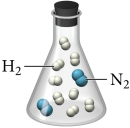# Problem: Nitrogen and hydrogen gas react to form ammonia according to the reaction:N2(g) + 3H2(g) → 2NH3(g)If a flask contains a mixture of reactants represented by the image at the far left, which image below best represents the mixture in the flask after the reactants have reacted as completely as possible?

###### FREE Expert Solution

We’re being asked to determine the image that represents that product that will be formed in the reaction

The given balanced reaction is:

N2(g) + 3H2(g) → 2 NH3(g)

To solve this problem, we need to:

Step 1. Determine the limiting reagent and the mole of NH3 produced.
Step 2. Determine the excess reagent remaining.

95% (319 ratings)###### Problem Details
Nitrogen and hydrogen gas react to form ammonia according to the reaction:

N2(g) + 3H2(g) → 2NH3(g)If a flask contains a mixture of reactants represented by the image at the far left, which image below best represents the mixture in the flask after the reactants have reacted as completely as possible?